Aniruddha Bhandari — July 20, 2020

## Introduction

Anything and everything related to data in the 21st century has become of prime relevance. And one of the key skills for any data science aspirant is mastering SQL functions for effective and efficient data retrieval. SQL is widely used for querying directly from databases and is, therefore, one of the most commonly used languages for data analysis tasks. But it comes with its own intricacies and nuances.When it comes to SQL functions, there are a plethora of them. You need to know the right function at the right time to achieve what you are looking for. But the majority of us including me have a tendency to skip this topic or keep it hanging till a distant future. And trust me it is a blunderous mistake to leave these topics unturned in your learning journey.

Therefore, in this article, I will take you through some of the most common SQL functions that you are bound to use regularly for your data analysis tasks.

If you are interested in learning SQL in a course format, please refer to our course – Structured Query Language (SQL) for Data Science

• Introducing the Dataset
• Aggregate functions in SQL
• Count
• Sum
• Average
• Min and Max
• Mathematical functions in SQL
• Absolute
• Ceil and Floor
• Truncate
• Modulo
• String functions in SQL

• Lower and Upper
• Concat
• Trim
• Date and Time functions in SQL
• Date and Time
• Extract
• Date format
• Windows functions in SQL
• Rank
• Percent value
• Nth value
• Miscellaneous functions
• Convert
• Isnull
• If

## Introducing the Dataset

I will show you the practical application of all the functions covered in this article by working with a dummy dataset. Let’s assume there is a retail chain all over the country. The following SQL table records who bought items from the retail shop, on what date they bought the item, the city they are from, and the purchase amount.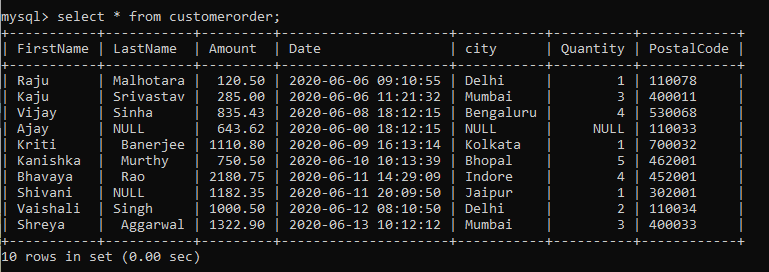We are going to use this example we learn the different functions in this article.

## Aggregate functions

• #### Count

One of the most important aggregate functions is the count() function. It returns the number of records from a column in the table. In our table, we can use the count() function to get the number of cities where the order came from. We do that as follows: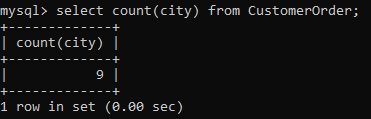You would have noticed two things here. Firstly, the Null function counts the null values. Then, duplicate values are counted multiple times. To deal with this problem, we can pair it with the distinct() function which will count only the distinct values in the column.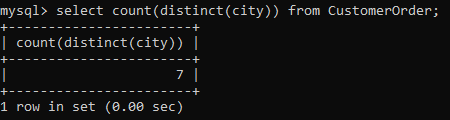• #### Sum

Whenever we are dealing with columns related to numbers, we are bound to check out their total sum. For example in our table, the total sum of Amount is important to analyze the sales that occurred.

The sum can be calculated using the sum() function which works on the column name.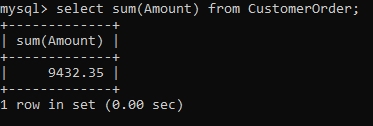But what if we want to calculate the total amount for every city?

For that to happen, we can combine this function with the Groupby clause to group the output by the city. Here is how you can make it happen.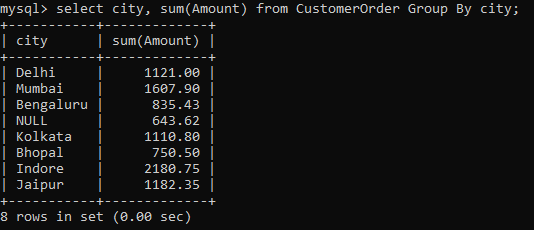This shows us that the company had Indore as the highest income generating city for us.

• #### Average

Anyone who has done some data analysis in the past knows that average is a better metric than just computing the sum of the numerical values.
In our example, we have multiple orders from the same city, therefore, it would be more prudent to calculate the average amount rather than the total sum.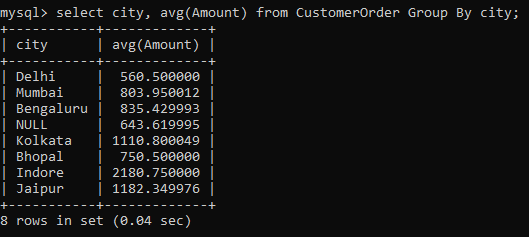• #### Min and Max

Finally, aggregate value analysis isn’t complete without computing the min and max values. These can be simply computed using the min() and max() functions.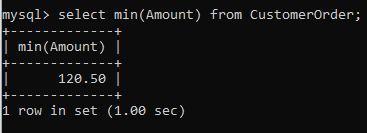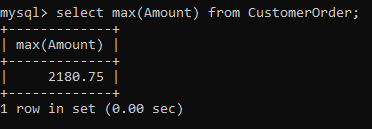## Mathematical functions

Most of the time you would have to deal with numbers in the SQL table for data analysis. To deal with these numbers, you need mathematical functions. These might have a trivial definition but when it comes to the analysis, they are the most prolifically used functions.

• #### Absolute

abs() is the most common mathematical function. It calculates the absolute value of a numeric value that you pass as an argument.

To understand where it is helpful, let’s first find out the deviation of the amount for every record from the average amount from our table.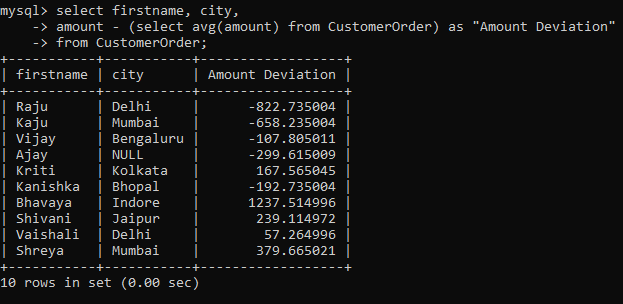Now, as you can see we have some negative values here. These can be easily converted to positives using the abs() function as shown below: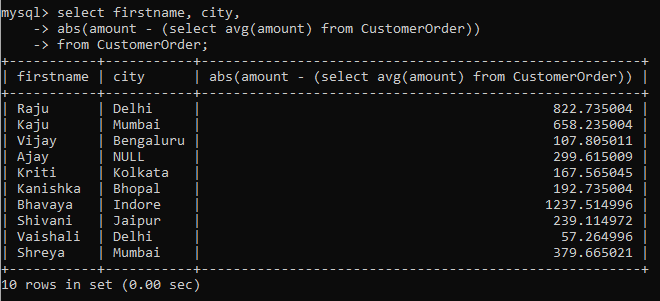• #### Ceil and Floor

When dealing with numeric values, some of them might have decimal values. How do you deal with those? You can simply convert them to either the next higher integer using ceil() or the previous lower integer using floor().
In our table, the Amount column has lots of decimal values. We can convert them to integers using ceil() or the floor() function.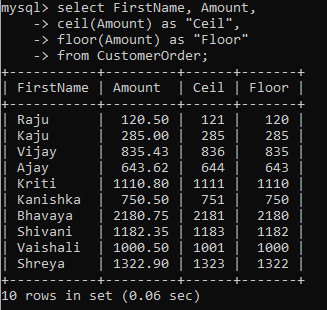• #### Truncate

Sometimes you would not want to convert a decimal value to an integer but truncate the number of decimal places in the number. Truncate() function achieves it. All you have to do is pass the decimal number as the first argument and the number of places you want to truncate it to as the second argument.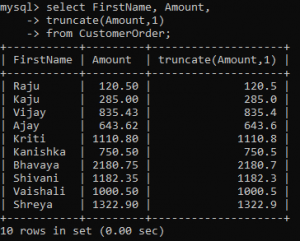As you can see, I have truncated the values to one decimal place.

• #### Modulo

The modulo function is a powerful and important function. Modulo returns the remainder left when the second number divides the first number. It is used by calling the function mod(x,y) where the result is the remainder left when x is divided by y.

It has a very important function in the analysis. You can use it to find the odd or even records from the SQL table. For example, in our example table, I can use modulo function to find those records which had an odd number of quantities.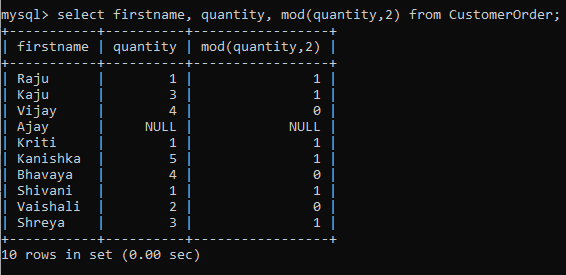Or I could find even quantities if I negate the above result by using the not keyword.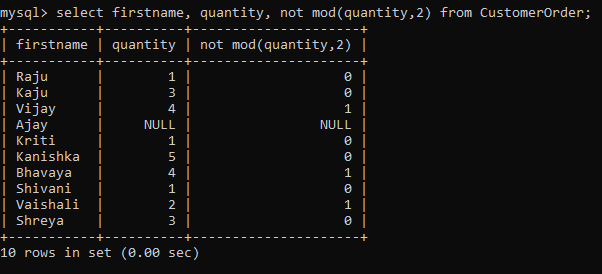## String functions

When you are working with SQL tables, you will have to deal with strings all the time. They are especially important when you want to output the result in a presentable way.

• #### Lower and Upper

You can convert the string values to uppercase or lowercase by using the upper() or lower() functions respectively. In short, this helps in bringing more consistency to the record values.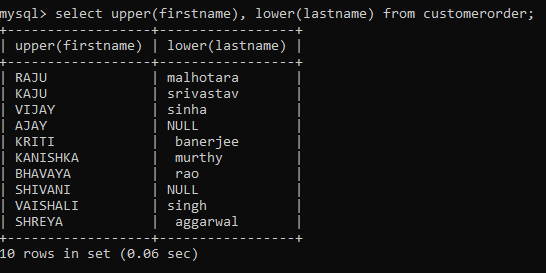• #### Concat

concat() function joins two or more strings into one. All you have to do is provide as argument the strings you want to concatenate.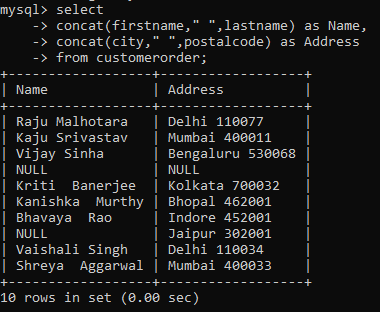As you would have noticed, even if one of the values is Null, the whole output is returned as a Null value.

• #### Trim

Trim is a very important function not just in SQL, but in any language there is. It is one of the most important string functions. It removes any leading or trailing whitespace from the string. For example, in our sample table, there are many trailing and leading whitespaces in the lastname column. We can remove these using the trim() function.

•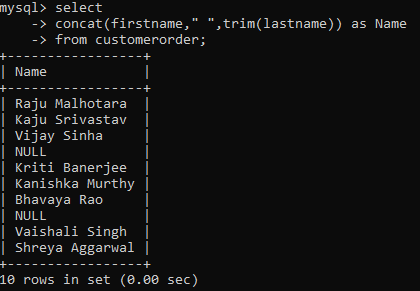As you can see, the function has trimmed any leading or trailing whitespaces from the string.

## Date and time functions

To begin with, there is no doubt about the relevance of date and time features. But this is only the case if you know how to handle them well! Check out the following date and time functions to master your analysis skills.

• #### Date and Time

If you have a common column for date and time as I have in the sample table, then you will need to use the date() and time() functions to extract the respective values.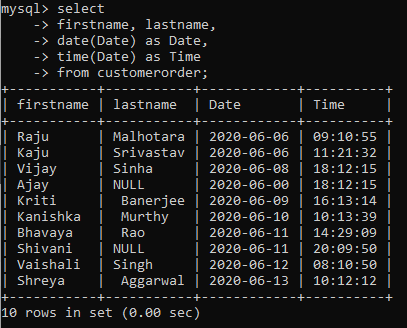• #### Extract

But sometimes you might want to go a step further and analyze how many of the orders were placed on a particular day of the week or month, or maybe the time of the day. For that, you need to use the super convenient extract() function.

The syntax is simple: extract(unit from date)

The unit can be anything from year, month, to a minute, or second.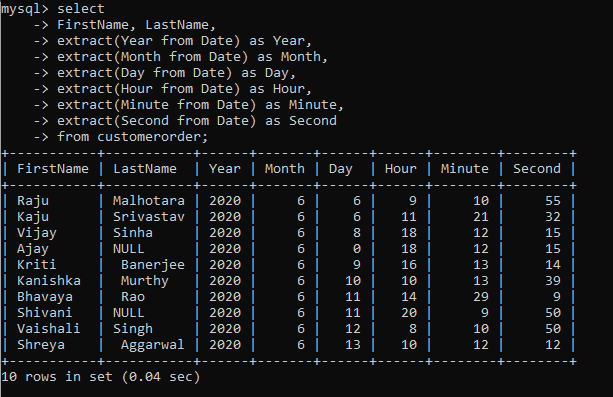You can even extract the week of the year or the quarter of the year.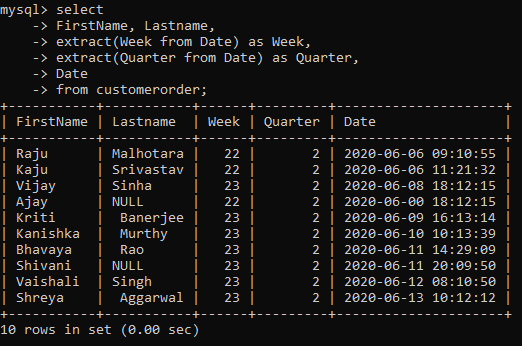A complete list of all the units that you can extract from the date is as follows: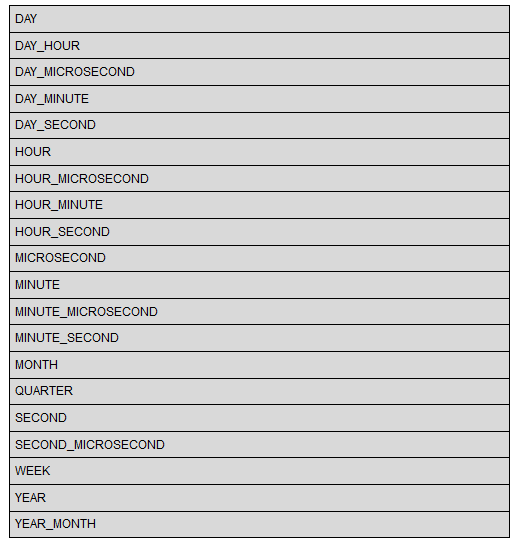As you can see, there is a lot of analysis that you can do with the extract() function!

• #### Date format

Sometimes the dates in the database will be saved in a different format compared to how you would want to view them. Therefore, to change the date format, you can use the date_format() function. The syntax is as follows: date_format(date, format)

Currently, the dates saved in the sample table are in the year-month-day format. Using this function, I will output the dates in the day-month name-year format.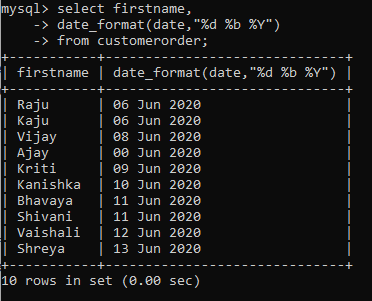There are a lot of opportunities to change the format according to your requirements. You can find all the format at this link.

## Windows functions

Window functions are important functions but can be tricky to understand. Therefore, we first start by understanding the basic window function.

#### Window function

A window function performs calculation similar to an aggregate function, but with a slight twist. While the regular aggregate functions group the rows into a single output value, window function does not do that. The window function works on a subset of rows but does not reduce the number of rows. The rows retain their individual identity. To understand it better, let’s compare a simple aggregate function sum().Here, we get the aggregate value of all the rows. Now let’s use the windows function for this aggregate function and see what happens.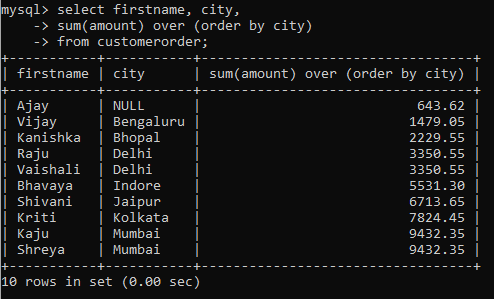As you must have noticed, we still get the aggregate sum values but they are segregated by the different city groups. Notice that we calculate the output for every row.

The OVER  clause turns the simple aggregate function into a windows function. The syntax is simple and as follows:

window_function_name(<expression>) OVER ( <partition_clause> <order_clause>)

The part before the OVER clause is the aggregate function or a windows function. We will cover a few window functions in the subsequent sections.

The part after the OVER clause can be divided into two parts:

• Partition_clause defines the partition between rows. The window function operates within each partition. The Partition by clause defines it.
• Order_clause orders the rows within the partition. The Order by clause defines it.

We will explore these in detail when we explore a few more window functions in the subsequent sections.

• #### Rank

The simple window function is the rank() function. As the name suggests, it ranks the rows within a partition group based on a condition.

It has the following syntax: Rank() Over ( Partition by <expression> Order By <expression>)

Let’s use this function to rank the rows in our table based on the amount of order within each city.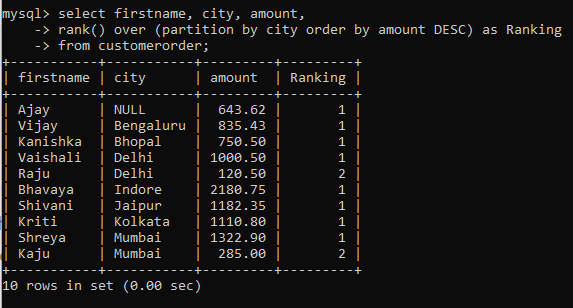Consequently, the rows have been ranked within their respective partition group (or city).

• #### Percent_value

It is an important window function that finds the relative rank of a row within a group. It determines the percentile value of each row.

Its syntax is as follows: Percent_rank() Over(Partition by <expression> Order by <expression>)

Although the partition clause is optional.

Let’s use this function to determine the amount percentile of each customer in the table.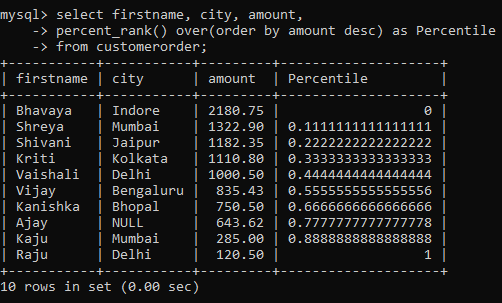• #### Nth_value

Sometimes you want to find out which row had the highest, lowest, or the nth highest value. For example, the highest scorer in school, top sales performer, etc. is in situations like these where you need the nth_value() windows function.

As a result, the function returns nth row value from an ordered set of rows. The syntax is as follows:

nth_value() order (partition by <expression> order by <expression>)

Let’s use this function to find out who was the top buyer in the table.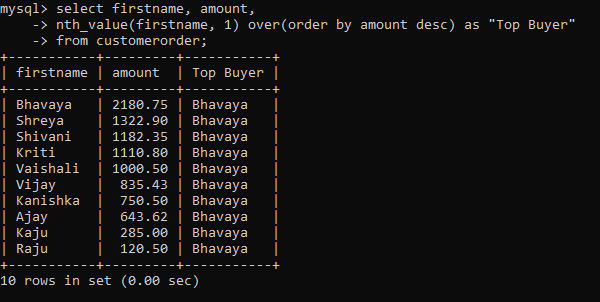## Miscellaneous functions

So far we have discussed very specific functions. Now we will explore some miscellaneous functions that can’t be categorized within a specific functional group but are of immense value.

• #### Convert

Sometimes you would want to convert the output value into a specified datatype. Moreover, you can think of it like casting, where you can change the data type of the value. Its syntax is simple: convert(value, type)

We can use it to convert the data type of the date column before we print the value.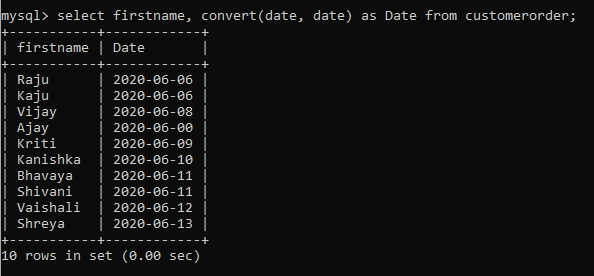• #### Isnull

Generally, if you don’t specify the non-value for your attribute, chances are you will end up with some null values in the column. But you can easily deal with them using the isnull() function.

You just have to write the expression within the function. It will return 1 for a null and 0 otherwise.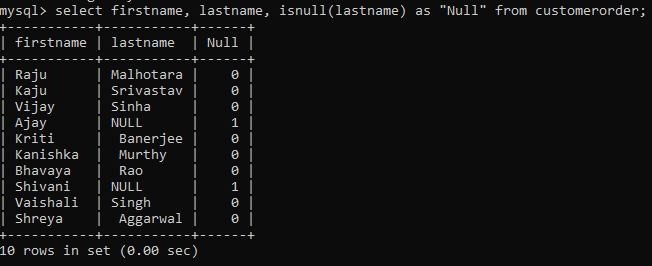Looks like we have some null values for lastname attribute in the table!

• #### If

Finally, the most important function you will ever use in SQL is the if() function. It lets you define the if-conditionality which you encounter in any programming language.

It has a simple syntax: if(expression, value_if_true, value_if_false)

Using this function, let’s find out which customer paid more than 1000 amount for their order.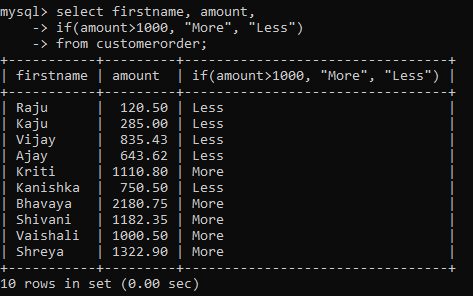Moreover, the use of this function is boundless and it is rightly used regularly for data analysis tasks.

## Endnotes

To summarize, we have covered a lot of basic SQL functions that are bound to be used quite a lot in day to day data analysis tasks. You may further broaden the application of some of the functions by reading the following article-

If you are interested in learning SQL in a course format, please refer to our course – Structured Query Language (SQL) for Data Science

Hope this article helps you bring out more from your dataset. And if you have any favorite SQL function that you find useful or use quite often, do comment below and share your experience!